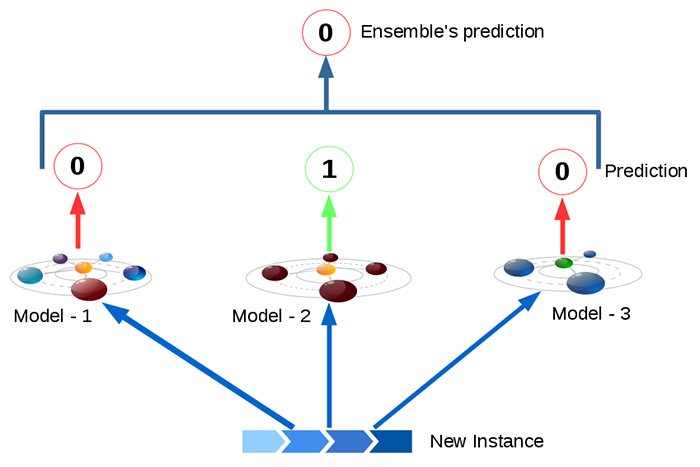# Heterogeneous Ensemble Learning (Hard voting / Soft voting)

### Voting Classifier

• Suppose you have trained a few classifiers, each one individually achieving about 80% accuracy (Logistic Regression classifier, an SVM classifier, a Random Forest classifier, a K-Nearest Neighbors classifier). We can create a better classifier by aggregating the predictions of each classifier and predict the class that gets the most votes. This approach is called as Voting Classification.

Hard Voting Classifier : Aggregate predections of each classifier and predict the class that gets most votes. This is called as “majority – voting” or “Hard – voting” classifier.Soft Voting Classifier : In an ensemble model, all classifiers (algorithms) are able to estimate class probabilities (i.e., they all have predict_proba() method), then we can specify Scikit-Learn to predict the class with the highest probability, averaged over all the individual classifiers.

Modle Name Class – 1 Probability Class – 0 Probability
Model – 1 0.49 0.51
Model – 2 0.99 0.01
Model – 3 0.49 0.51
Averages 0.66 0.34

This soft-voting classifier often work better than hard-voting as it gives more weight to highly confident votes. Need to specify voting=”soft” and ensure that all classifiers can estimate class probabilities.

• One algorithm where we need to be careful is SVC, by default SVC will not give probabilities, we have to specify “probability” hyperparameter to True.

In :

```import pandas as pd
import numpy as np
import matplotlib.pyplot as plt
% matplotlib inline
import seaborn as sns
from sklearn.model_selection import train_test_split
plt.rcParams['axes.labelsize'] = 14
plt.rcParams['xtick.labelsize'] = 12
plt.rcParams['ytick.labelsize'] = 12
import warnings
warnings.filterwarnings('ignore')
import random
random.seed(10)
```

In :

```from sklearn.model_selection import train_test_split
from sklearn.datasets import make_moons
X, y = make_moons(n_samples=500, noise=0.30, random_state=42)
X_train, X_test, y_train, y_test = train_test_split(X, y, random_state=42)
```

### Hard Voting

In :

```from sklearn.ensemble import RandomForestClassifier
from sklearn.ensemble import VotingClassifier
from sklearn.linear_model import LogisticRegression
from sklearn.svm import SVC
log_clf = LogisticRegression(random_state=42)
rnd_clf = RandomForestClassifier(random_state=42)
svm_clf = SVC(random_state=42)
hard_voting_clf = VotingClassifier(
estimators=[('lr', log_clf), ('rf', rnd_clf), ('svc', svm_clf)],
voting='hard')
hard_voting_clf.fit(X_train, y_train)
```
```Out : VotingClassifier(estimators=[('lr', LogisticRegression(C=1.0, class_weight=None, dual=False, fit_intercept=True,
intercept_scaling=1, max_iter=100, multi_class='ovr', n_jobs=1,
penalty='l2', random_state=42, solver='liblinear', tol=0.0001,
verbose=0, warm_start=False)), ('rf', RandomFor...f',
max_iter=-1, probability=False, random_state=42, shrinking=True,
tol=0.001, verbose=False))],
flatten_transform=None, n_jobs=1, voting='hard', weights=None)```

In :

```from sklearn.metrics import accuracy_score
for clf in (log_clf, rnd_clf, svm_clf):
clf.fit(X_train, y_train)
y_pred = clf.predict(X_test)
print(clf.__class__.__name__, accuracy_score(y_test, y_pred))
hvc_predict = hard_voting_clf.predict(X_test)
print("Hard voting clasifier accuracy: ", accuracy_score(y_test, hvc_predict))
```
`Out :`
`LogisticRegression 0.864`
```RandomForestClassifier 0.872
SVC 0.888
Hard voting clasifier accuracy:  0.896```

### Soft Voting

In :

```log_clf = LogisticRegression(random_state=42)
rnd_clf = RandomForestClassifier(random_state=42)
svm_clf = SVC(probability=True, random_state=42)
soft_voting_clf = VotingClassifier(
estimators=[('lr', log_clf), ('rf', rnd_clf), ('svc', svm_clf)],
voting='soft')
soft_voting_clf.fit(X_train, y_train)
```
`Out :`
```VotingClassifier(estimators=[('lr', LogisticRegression(C=1.0, class_weight=None, dual=False, fit_intercept=True,
intercept_scaling=1, max_iter=100, multi_class='ovr', n_jobs=1,
penalty='l2', random_state=42, solver='liblinear', tol=0.0001,
verbose=0, warm_start=False)), ('rf', RandomFor...bf',
max_iter=-1, probability=True, random_state=42, shrinking=True,
tol=0.001, verbose=False))],
flatten_transform=None, n_jobs=1, voting='soft', weights=None)```

In :

```from sklearn.metrics import accuracy_score
for clf in (log_clf, rnd_clf, svm_clf, soft_voting_clf):
clf.fit(X_train, y_train)
y_pred = clf.predict(X_test)
print(clf.__class__.__name__, accuracy_score(y_test, y_pred))
```
`Out :`
```LogisticRegression 0.864
RandomForestClassifier 0.872
SVC 0.888
VotingClassifier 0.912```
Categories:DataJango - Chatbot